# 978-0134292380 Chapter 10

Document Type
Test Prep
Book Title
Fundamentals of Hydraulic Engineering Systems 5th Edition
Authors
A. Osman H. Akan, Ned H. C. Hwang, Robert J. Houghtalen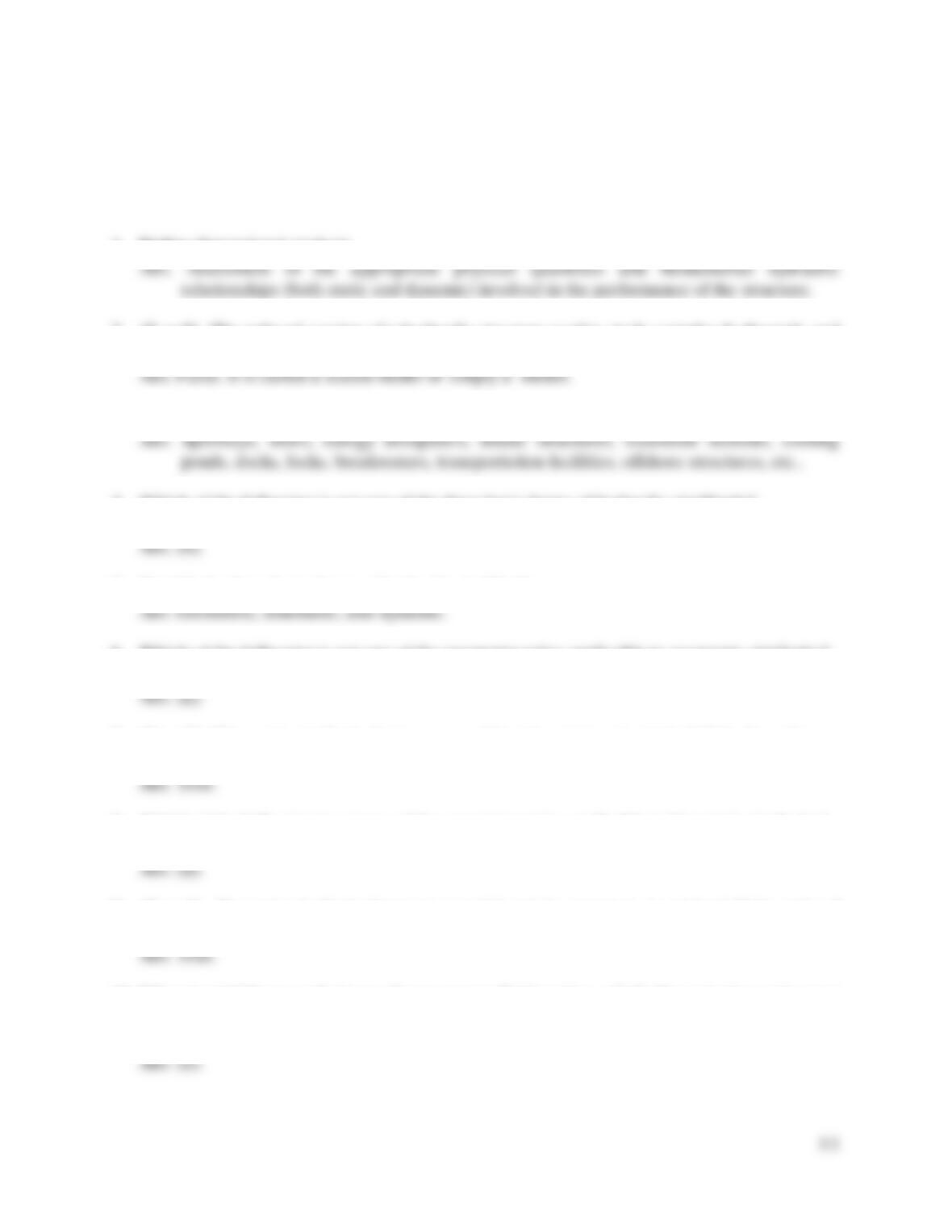93
TEST QUESTIONS & PROBLEMS - CHAPTER #10
1. Define dimensional analysis.
2. (T or F) The reduced version of a hydraulic structure used to study complex hydrostatic and
hydrodynamic phenomena is called a prototype.
3. Name three hydraulic structures that are designed or analyzed with the help of model studies.
4. Which of the following is not one of the three basic forms of hydraulic similitude?
a) geometric b) kinetic c) kinematic d) dynamic
5. Identify the three basic forms of hydraulic similitude.
6. Which of the following is not one of the parameter ratios applicable to geometric similarity?
a) velocity b) length c) area d) volume
7. (T or F) Kinematic similarity between a model and prototype is attained if the homologous
moving particles have the same velocity ratio along geometrically similar paths.
8. Which of the following is not one of the parameter ratios applicable to kinematic similarity?
a) angular acceleration b) discharge c) velocity d) mass e) time
9. (T or F) Dynamic similarity between a model and its prototype is attained if the ratio of
homologous forces (prototype to model) is kept at a constant value.
10. When inertial forces and viscous forces govern fluid motion, which dimensionless ratio must
be maintained between the model and the prototype??
a) Reynolds number b) Froude number c) Weber number d) Social Security number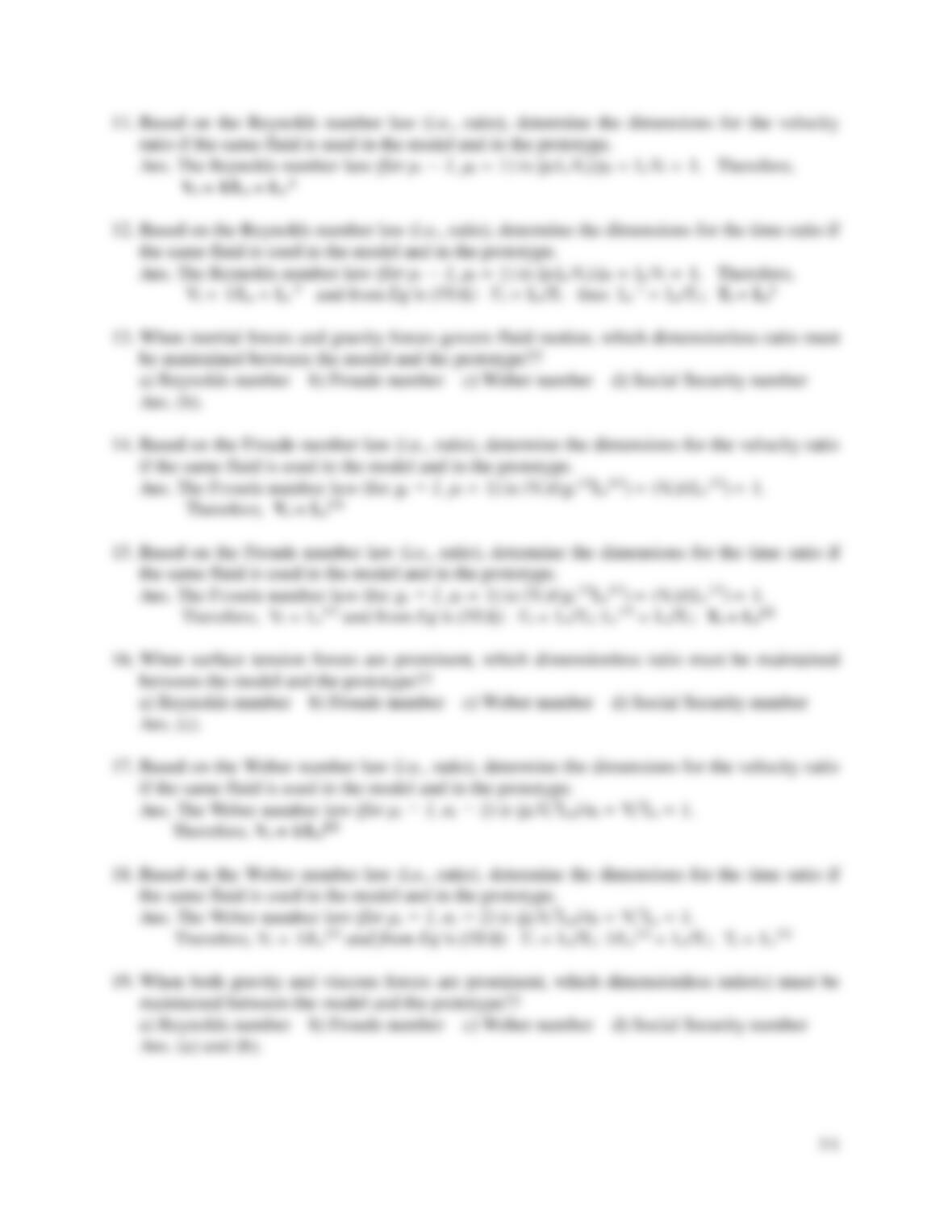11. Based on the Reynolds number law (i.e., ratio), determine the dimensions for the velocity
12. Based on the Reynolds number law (i.e., ratio), determine the dimensions for the time ratio if
13. When inertial forces and gravity forces govern fluid motion, which dimensionless ratio must
be maintained between the model and the prototype??
a) Reynolds number b) Froude number c) Weber number d) Social Security number
14. Based on the Froude number law (i.e., ratio), determine the dimensions for the velocity ratio
15. Based on the Froude number law (i.e., ratio), determine the dimensions for the time ratio if
16. When surface tension forces are prominent, which dimensionless ratio must be maintained
between the model and the prototype??
a) Reynolds number b) Froude number c) Weber number d) Social Security number
17. Based on the Weber number law (i.e., ratio), determine the dimensions for the velocity ratio
18. Based on the Weber number law (i.e., ratio), determine the dimensions for the time ratio if
19. When both gravity and viscous forces are prominent, which dimensionless ratio(s) must be
maintained between the model and the prototype??
a) Reynolds number b) Froude number c) Weber number d) Social Security number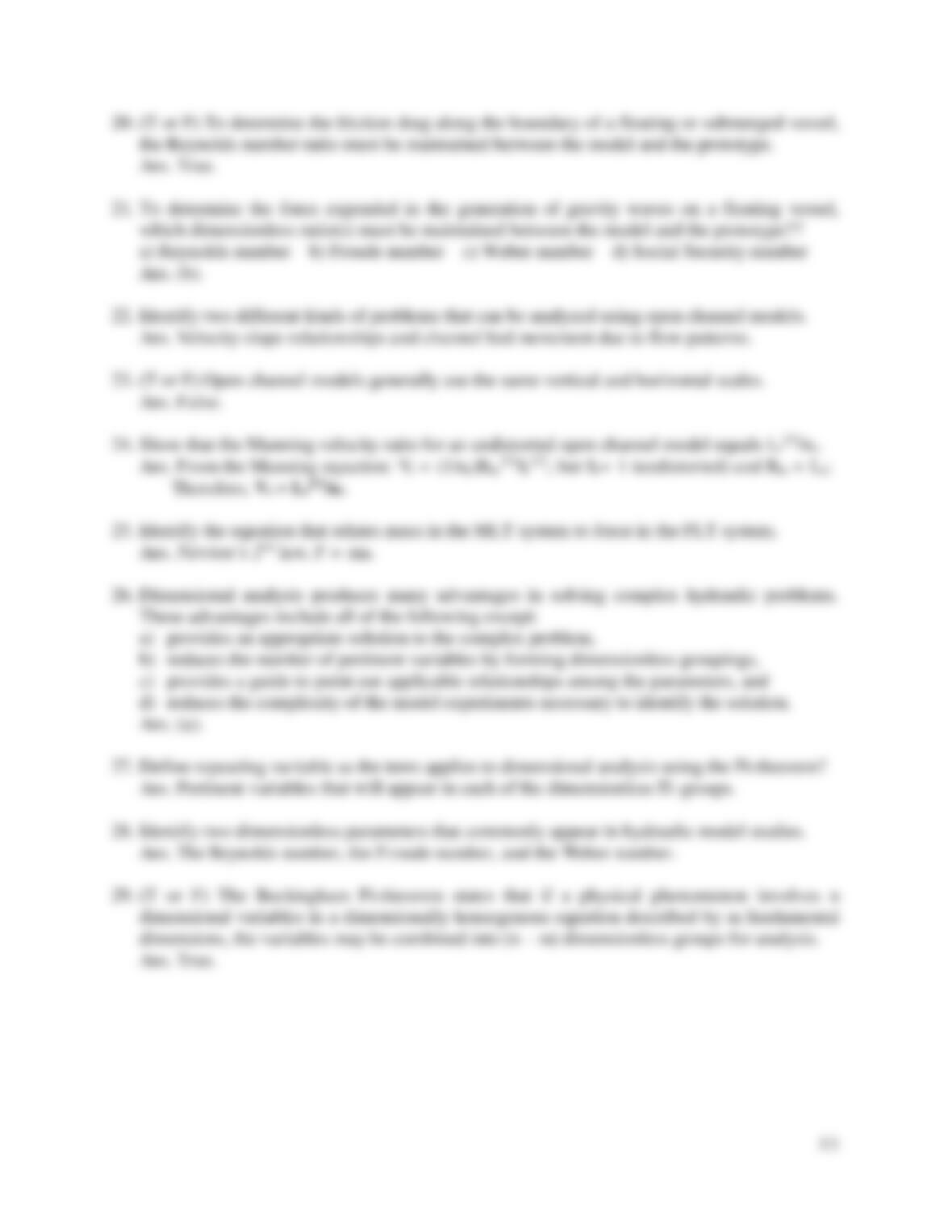20. (T or F) To determine the friction drag along the boundary of a floating or submerged vessel,
the Reynolds number ratio must be maintained between the model and the prototype.
21. To determine the force expended in the generation of gravity waves on a floating vessel,
which dimensionless ratio(s) must be maintained between the model and the prototype??
a) Reynolds number b) Froude number c) Weber number d) Social Security number
22. Identify two different kinds of problems that can be analyzed using open channel models.
Ans. Velocity-slope relationships and channel bed movement due to flow patterns.
23. (T or F) Open channel models generally use the same vertical and horizontal scales.
24. Show that the Manning velocity ratio for an undistorted open channel model equals Lr2/3/nr.
Ans. From the Manning equation: Vr = (1/nr)Rhr2/3Sr1/2; but Sr= 1 (undistorted) and Rhr = Lr;
Therefore, Vr = Lr2/3/nr
25. Identify the equation that relates mass in the MLT system to force in the FLT system.
Ans. Newton’s 2nd law, F = ma.
26. Dimensional analysis produces many advantages in solving complex hydraulic problems.
These advantages include all of the following except:
a) provides an appropriate solution to the complex problem,
b) reduces the number of pertinent variables by forming dimensionless groupings,
c) provides a guide to point out applicable relationships among the parameters, and
d) reduces the complexity of the model experiments necessary to identify the solution.
27. Define repeating variable as the term applies to dimensional analysis using the Pi-theorem?
28. Identify two dimensionless parameters that commonly appear in hydraulic model studies.
29. (T or F) The Buckingham Pi-theorem states that if a physical phenomenon involves n
dimensional variables in a dimensionally homogenous equation described by m fundamental
dimensions, the variables may be combined into (n m) dimensionless groups for analysis.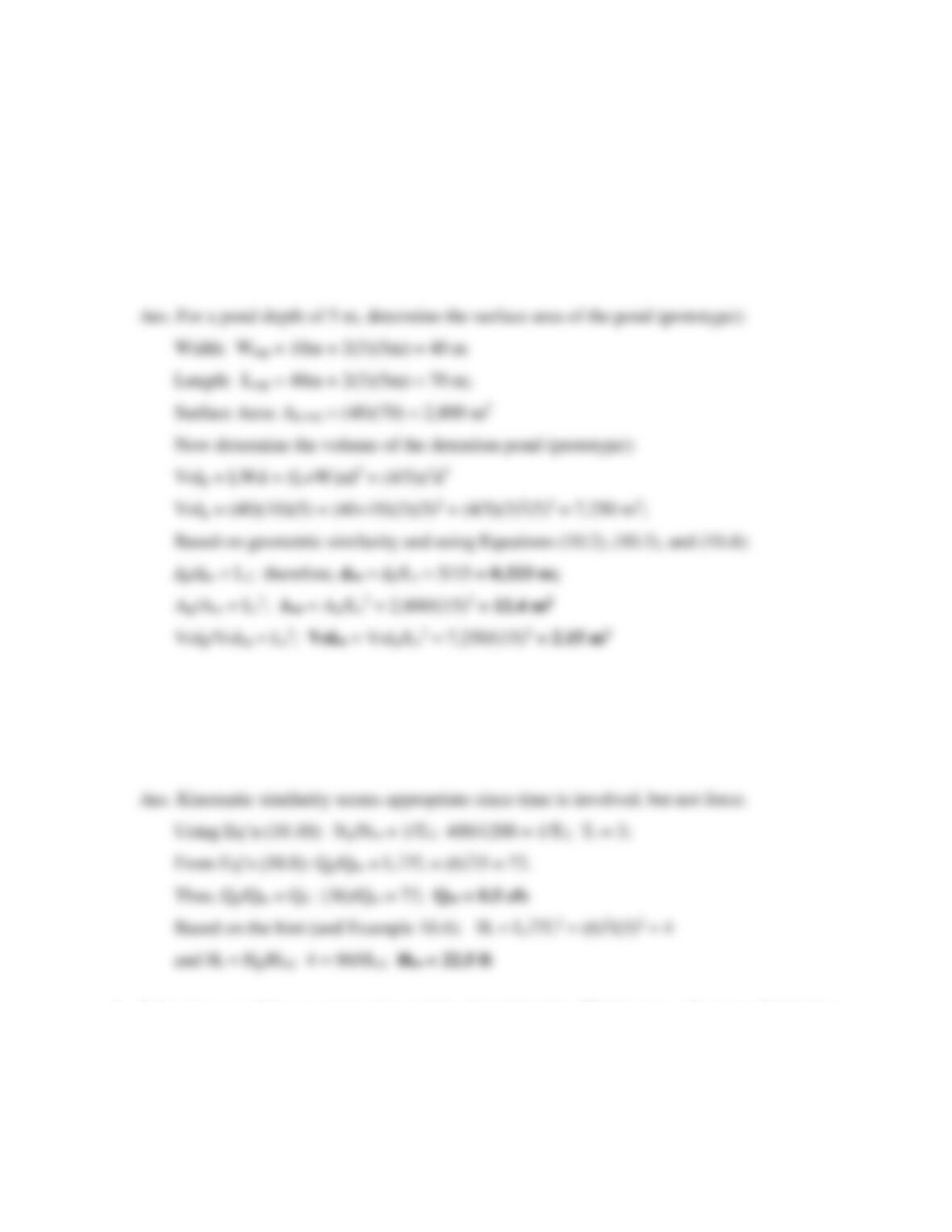96
Problems
1. A 1:15 scale model will be used to study circulation patterns in a rectangular detention pond.
The pond has a bottom length of 40 m and bottom width of 10 m. All sides of the pond have
3:1 (H:V) slopes. If the design depth is 5 m, determine the depth, surface area, and storage
volume of the model. (Note: The storage volume expression is: Vol = LWd + (L+W)zd2 +
(4/3)z2d3; where L, W, d, and z are the bottom length, bottom width, depth, and side slope.)
2. A centrifugal pump discharges 36 cfs and overcomes a 90-ft head when rotating at 400 rpm.
A 1:6 scale model operating at 1200 rpm is used to study the prototype. Find the model
discharge and head. (Hint: For hydraulic machines, head has the units of Lr2/Tr2 since it
incorporates the gravitational acceleration which is the same for model and prototype.)
3. Laboratory models are commonly used to determine the effectiveness of energy dissipation
structures. Consider a 1:20 scale model of an energy dissipator that is constructed to study
force distribution and water depths. A velocity ratio of 7.75 is used. Determine the force ratio
and prototype discharge if the model discharge is 10.6 cfs.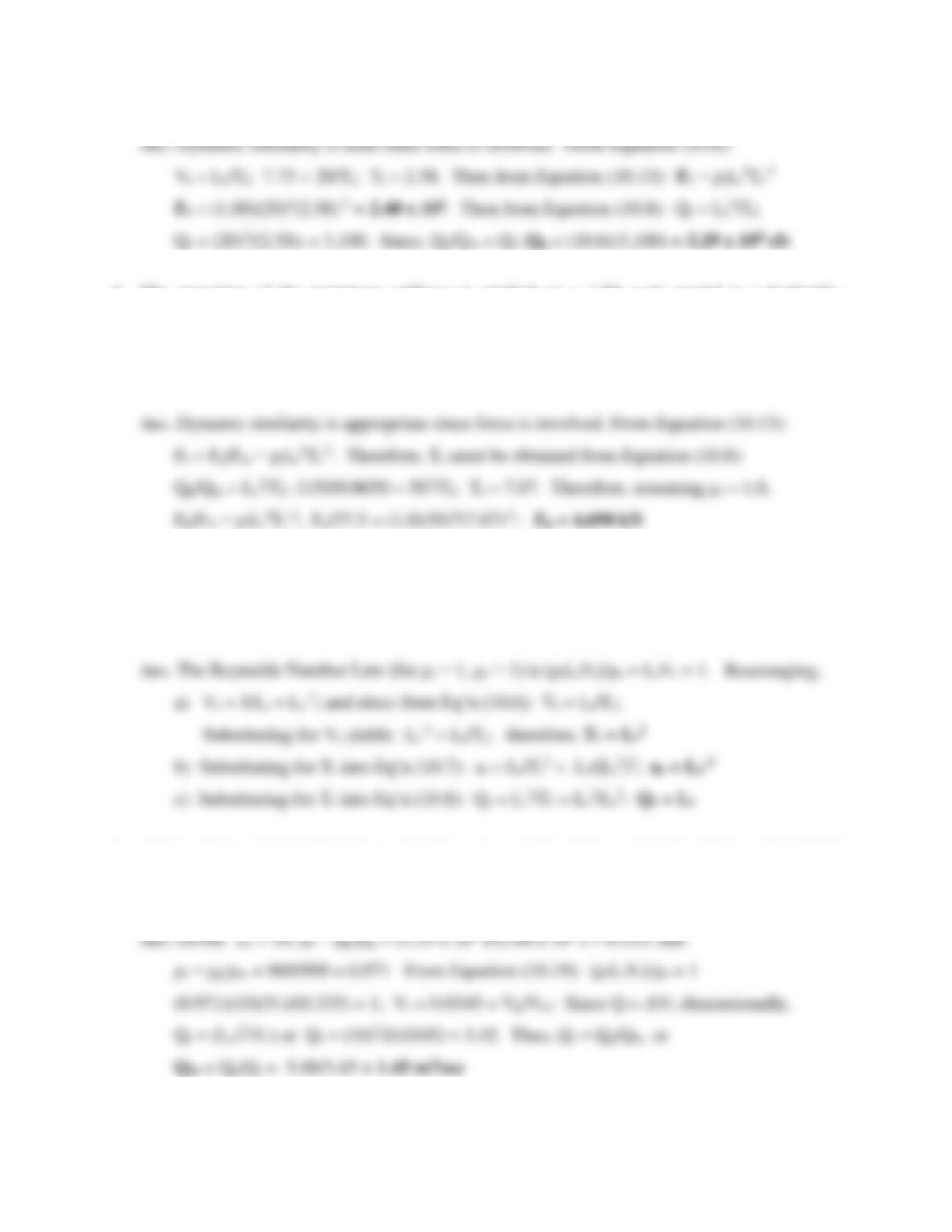97
4. The operation of the prototype spillway is studied on a 1:50 scale model in a hydraulic
laboratory. The prototype has a 100-m-long crest and conveys a design discharge of 1150
m3/sec under a permitted maximum head of 3.00 m. The flow rate in the model is 0.0650
m3/sec. If the force on a bucket energy dissipater at the toe of the spillway model is measured
to be 37.5 N, determine the corresponding force on the prototype.
5. For a particular model study, the inertial forces and viscous forces are known to govern the
motion. If the model and prototype both use water verify the scale ratios for:
a) time (Tr = Lr2), b) acceleration (ar = Lr-3), and c) discharge (Qr = Lr).
6. A Reynolds number 1:10 scale model of a water supply piping system is to be tested at 20°C
to find the head loss in the prototype that carries water at 85°C (ρp = 969 kg/m3; μ p = 3.35 x
10-4 N∙s/m2). The prototype is designed to carry 5.0 m3/sec. Determine the model discharge.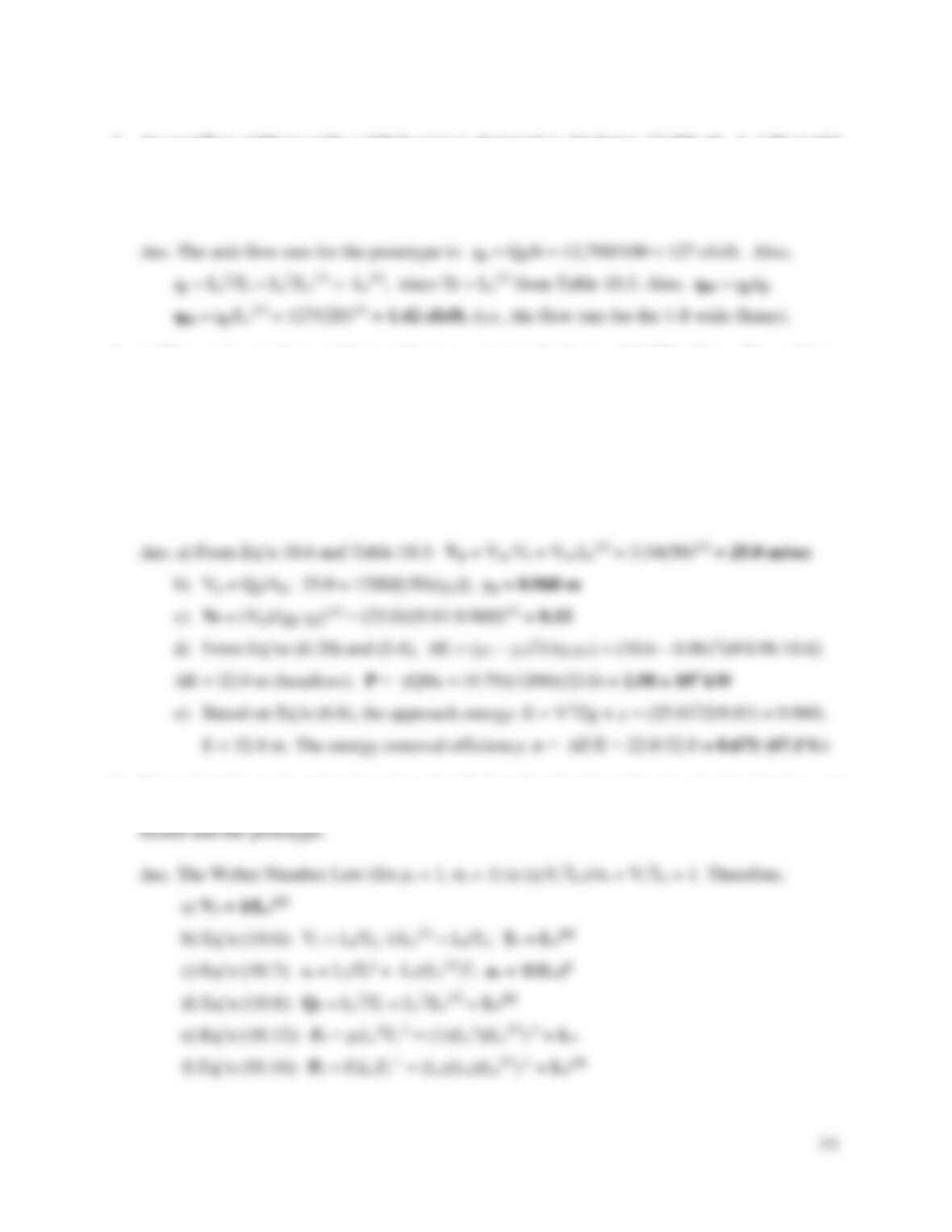98
7. An overflow spillway with a 100-ft crest is designed to discharge 12,700 cfs. A 1:20 model
of a portion of the cross section of the dam is built in a laboratory flume 1 ft wide. Calculate
the required laboratory flow rate assuming viscosity and surface tension effects are negligible
(i.e., inertial and gravity forces dominate).
8. A 50-m-wide overflow spillway will carry a design discharge of 1,200 m3/sec. The spillway
operation is studied on a 1:50 scale model in a hydraulic laboratory assuming inertial and
gravity forces are dominant. Determine the following prototype characteristics:
(a) The velocity if the spillway toe velocity in the model is 3.54 m/sec.
(b) The depth at the bottom (toe) of the prototype spillway.
(c) The Froude number at the bottom (toe) of the prototype spillway.
(d) The energy dissipation (in kilowatts) if a hydraulic jump occurs with y2 = 10.6 m.
(e) The energy removal efficiency of the hydraulic jump (energy dissipater)?
9. Determine the scale ratios based on the Weber Number Law for a) velocity, b) time, c)
acceleration, d) discharge, e) force, and f) power. Assume the same liquid is used in the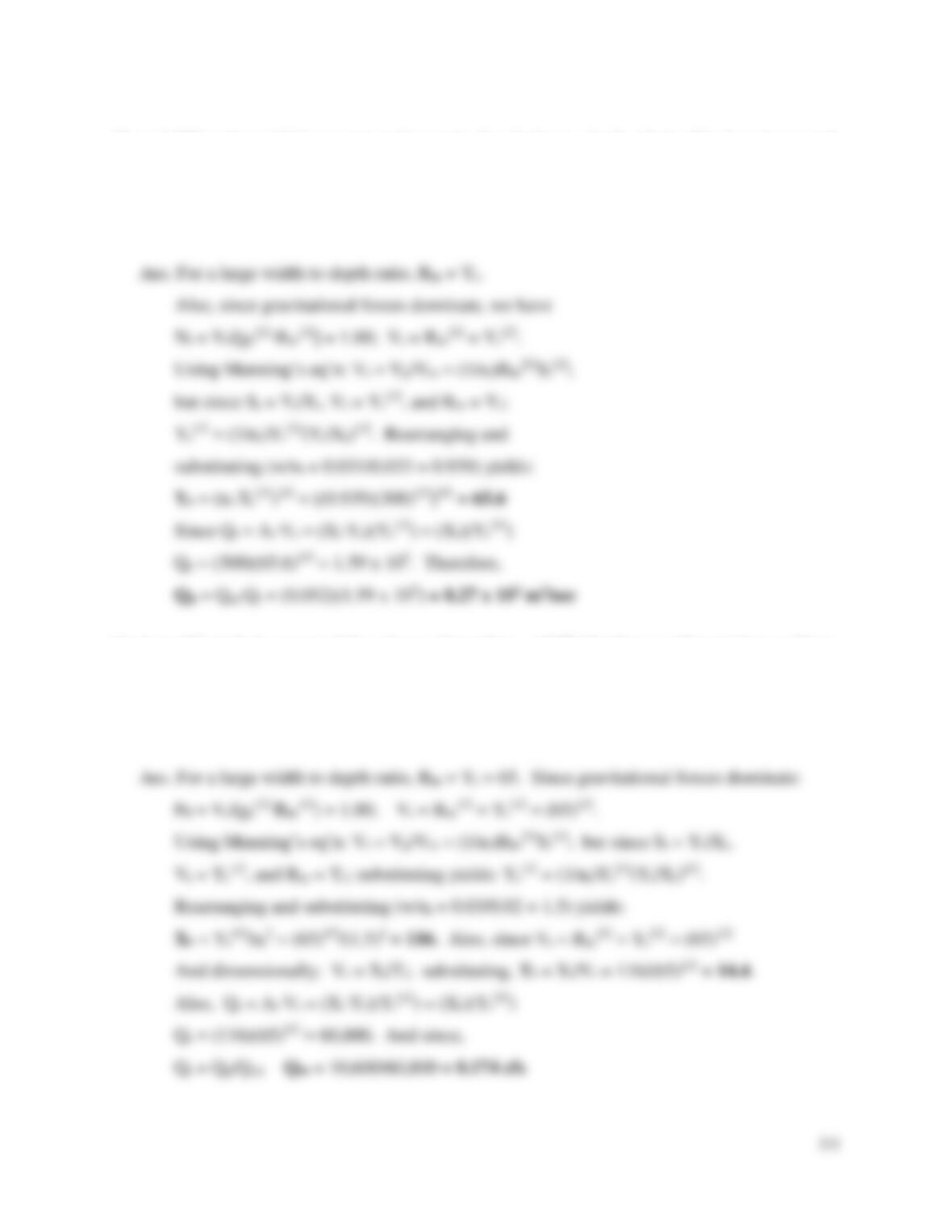10. A 1:300 scale model is constructed to study the discharge-depth relationship in a river reach
with Manning’s coefficient n = 0.031. If the model discharges 52ℓ/sec and has Manning’s
coefficient n = 0.033, determine the appropriate vertical scale ratio and the flowrate for the
prototype. (Assume the channel has a large width-to-depth ratio, and the hydraulic radius is
roughly equal to the water depth.)
11. A model study is proposed for a barge channel (np = 0.03) that has a sedimentation problem.
The channel conveys a flow rate of 10,600 cfs. The following model parameters have been
established: a vertical scale ratio of 1:65 and a roughness coefficient of nm = 0.02. Determine
the horizontal scale ratio, the time ratio, and the model flow. (Assume the channel has a large
width-to-depth ratio, and the hydraulic radius is roughly equal to the water depth.)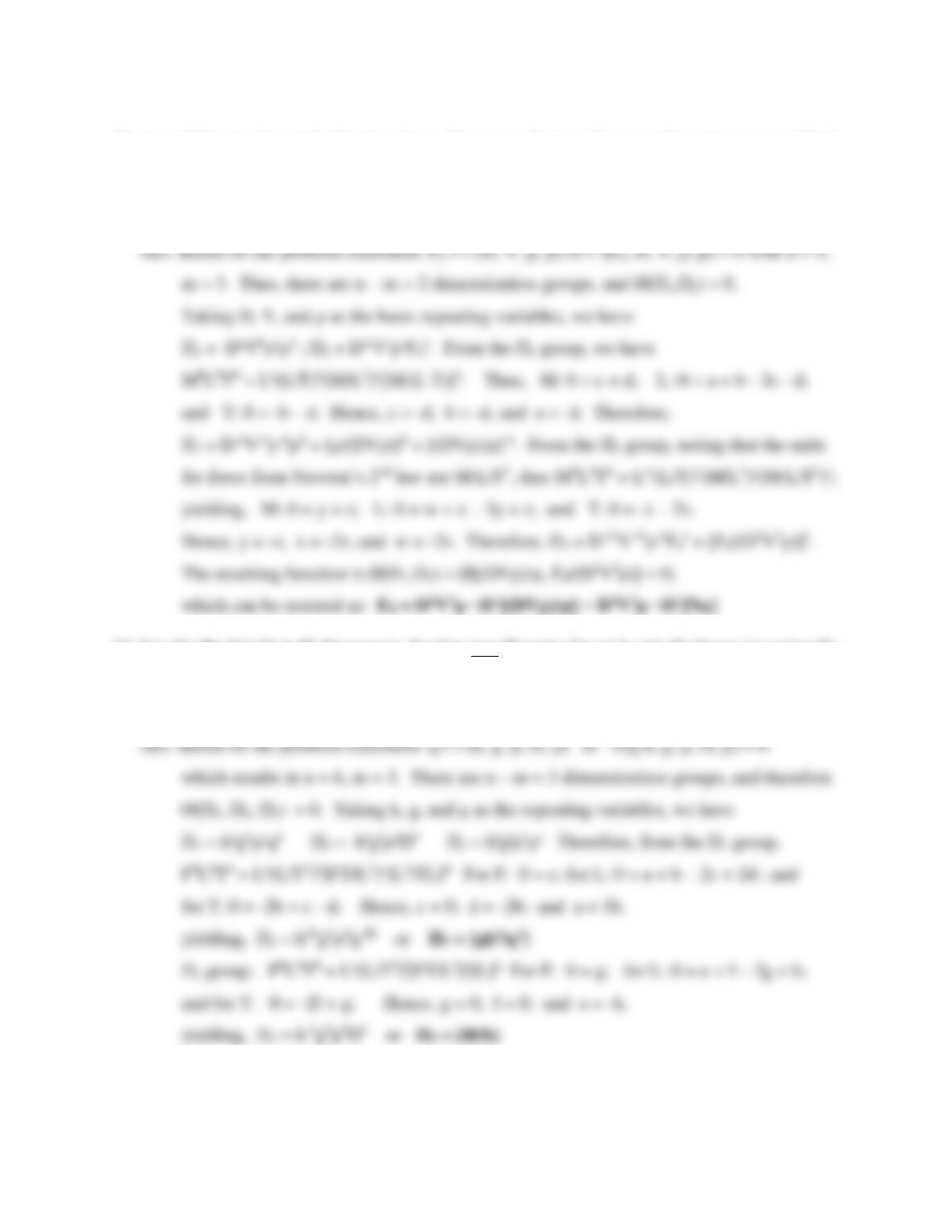12. A model is used to study the drag force (Fd) on a sphere as if moves through a viscous fluid.
The drag force is a function of sphere diameter (D), the sphere velocity (V), the viscosity of
the fluid (μ), and the fluid density (ρ). Use the Buckingham Pi-theorem (with diameter,
velocity, and density as repeating variables) to derive an expression for the drag force.
13. Use the Buckingham Pi theorem to develop two Pi terms for weir unit discharge (q) using the
following variables: spillway height (h), gravitational acceleration (g), viscosity (μ), head
(H), and density (ρ). Use h, g, and μ as repeating and the FLT system of units.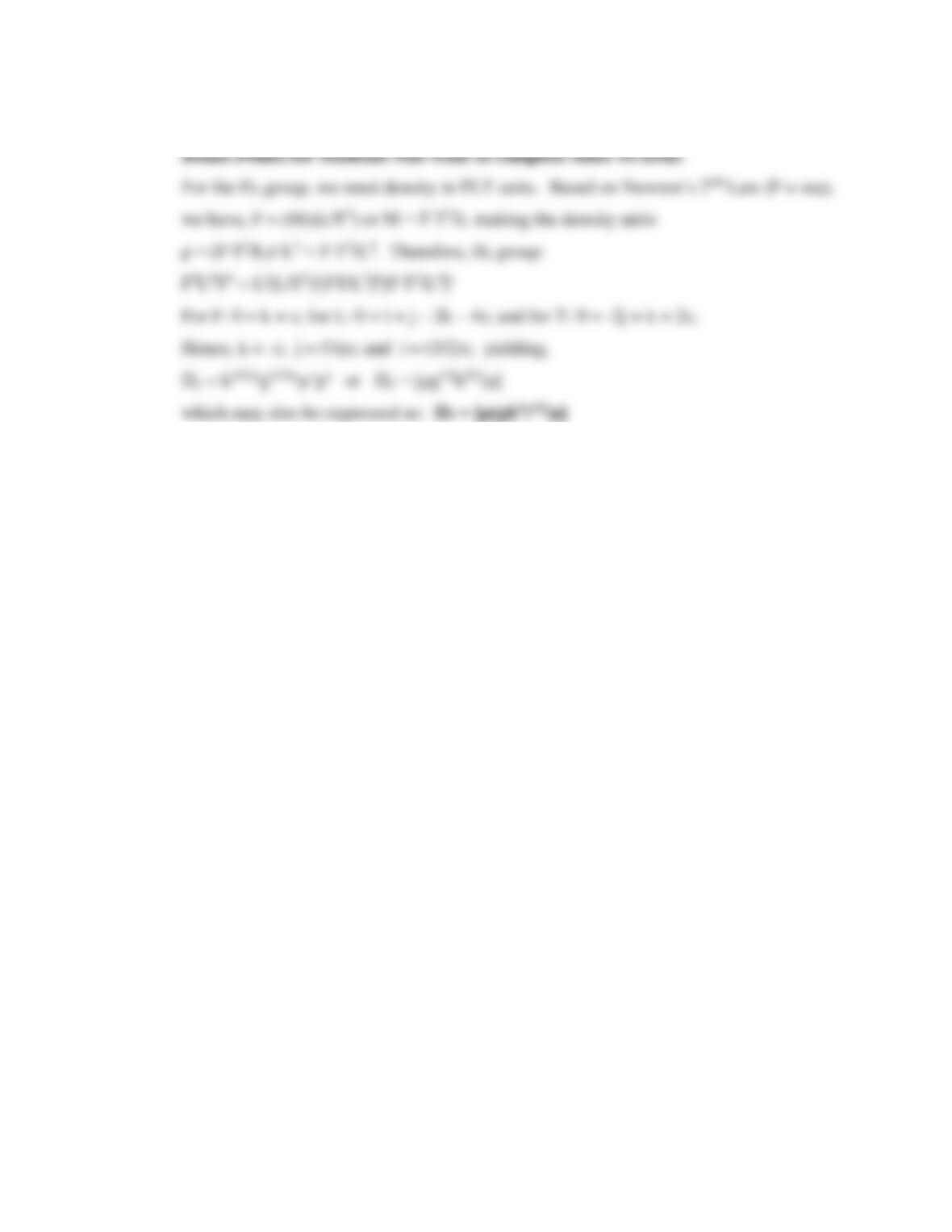101

## Trusted by Thousands ofStudents

Here are what students say about us.